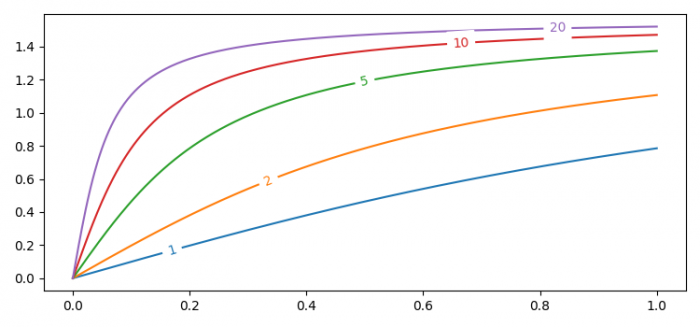# How can I draw inline line labels in Matplotlib?

To draw inline labels in Matplotlib, we can use labelLines() method. −

## Steps

• Set the figure size and adjust the padding between and around the subplots.
• Create random data points x using numpy and a list of data points, A.
• Iterate the list of A, and plot X and a (iterated item) with label.
• Label all the lines with their respective legends, for lines drawn.
• To display the figure, use show() method.

## Example

import numpy as np
from matplotlib import pyplot as plt
from labellines import labelLines

plt.rcParams["figure.figsize"] = [7.50, 3.50]
plt.rcParams["figure.autolayout"] = True

X = np.linspace(0, 1, 500)
A = [1, 2, 5, 10, 20]

for a in A:
plt.plot(X, np.arctan(a*X), label=str(a))

labelLines(plt.gca().get_lines(), zorder=2.5)

plt.show()

## Output